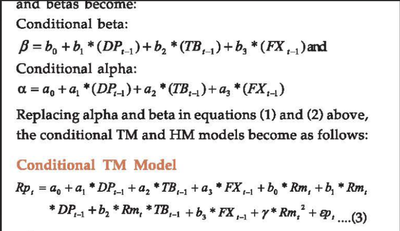## SAS conditional regressionI do not understand how conditional beta and alpha regression is done. How to regress the beta on betas and which model regress the alpha on alphas? The variable DP, TB and FX are Time series variables. I need help in understanding how we regress Time series conditional variables?

2 REPLIES 2

## Re: SAS conditional regression

[ I have moved this post to 'Forecasting and Econometrics' board ]

Hello @Sowmya_123 ,

Always a good idea to give a little bit of context when posing a question!
Are the shortcuts TM and HM referring to Treynor and Mazuy (1966) and Henriksson and Merton (1981)?

And are you dealing with stock markets (stock picking, stock pricing, funds, ...)?

So, you have regression coefficients changing over time.

Have you looked at what is known in econometric literature as :

• dynamic regression models
• random effects models (as opposed to fixed effects models)

?

Thanks,

Koen

## Re: SAS conditional regression

Hi Koen,

I was referring to both Treynor and Mazuy (1966) and Henriksson and Merton (1981) and I am working on Mutual fund performance in Recession and Expansion in the Technology sector.I am trying to do Ferson and Schadt(1996) conditional model.I don't have any econometrics background and know basic statistics. Hence I have trouble understanding the conditional model.

Discussion stats
• 2 replies
• 203 views
• 1 like
• 2 in conversation